# incidental

This tutorial provides a walkthrough on how to use incidental to estimate incidence. We will use the Spanish flu mortality data provided with this package.

library(incidental)
library(ggplot2)

## Data Format

The fit_incidence function requires two basic inputs:

• reported: a vector of the number of reported cases per day, and
• delay_dist: a probability vector that specifies the distribution of the number of days between infection and reporting.

The function assumes reported records are evenly spaced. Let’s examine how these values look for the flu data.

head(spanish_flu)
#>         Date Indiana Kansas Philadelphia
#> 1 1918-09-01       1      1            2
#> 2 1918-09-02       0      0            6
#> 3 1918-09-03       2      1            4
#> 4 1918-09-04       4      0            1
#> 5 1918-09-05       1      0            3
#> 6 1918-09-06       2      1            4
head(spanish_flu_delay_dist)
#>   days   proportion
#> 1    1 9.917752e-06
#> 2    2 3.376003e-03
#> 3    3 1.009925e-02
#> 4    4 2.292588e-02
#> 5    5 4.246682e-02
#> 6    6 6.261672e-02
delay_dist <- spanish_flu_delay_dist$proportion print(abs(sum(delay_dist) - 1)) #>  1.110223e-16 The data has daily mortality records for three locations (Indiana, Kansas, and Philadelphia). The delay distribution specifies the probability of a death occurring x days after infection. ## Model Fitting This package uses an empirical Bayes deconvolution method to fit an incidence curve from a series of recorded cases. The method uses a regularized Poisson likelihood function on a set of Poisson basis functions. We give basic use cases below. indiana_model = fit_incidence( reported = spanish_flu$Indiana,
delay_dist = delay_dist
)

kansas_model = fit_incidence(
reported = spanish_flu$Kansas, delay_dist = delay_dist ) philly_model = fit_incidence( reported = spanish_flu$Philadelphia,
delay_dist = delay_dist
)

## Model Outputs

The fitted incidence models have three outputs:

• Chat: an estimate of the noiseless case curve given the MAP incidence fit,
• Ihat: a MAP estimate of the incidence curve, and
• Isamps: a matrix of posterior samples from the incidence curve.

Let’s plot these outputs for Kansas. The data set also contains data for Indiana and Philadelphia. The function, incidence_to_df extracts the date (Date), the reported cases (Reported), the MAP incidence curve (Ihat), 90% pointwise credible interval bands around the incidence curve (CI_low, CI_high), and the implied smooth case curve (Chat), from a model and the data set that it was trained on. Another function, plot, plots these values.

plot(kansas_model, times = spanish_flu$Date)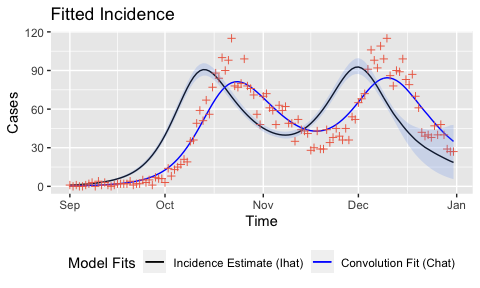# Advanced Usage The tunable parameters have been set so that they are robust for the majority of COVID-19 case records in the USA. This makes fits for influenza mortality data somewhat overregularized. More advanced settings include changing basis function degrees of freedom and regularization parameter selection methods, and extrapolation parameters for right censoring. We note, however, that this method is sensitive to bad data in the last two to three weeks of the time series (zero counts, counts that are too high, etc). We recommend either cleaning that data or not using the incidence estimates from that period. ## Linear Extrapolation This package uses a Bayesian linear model as a mean function for an AR extrapolation to cope with right censoring. There are two parameters that control how that fit is conducted: • linear_tail: an integer number of days used to fit linear model on tail to be used as a mean for AR extrapolation (default 14); and • extrapolation_prior_precision: a positive scalar for extrapolation slope shrinkage prior precision (default 2). These values control right tail fits. Let’s try a few combinations on the Kansas data using the functions that we made above. linear_tail_values = c(7, 14, 21) extrapolation_prior_precision_values = c(0, 10, 100) model_df_list = list() counter = 1 for (linear_tail_value in linear_tail_values) { for (extrapolation_prior_precision_value in extrapolation_prior_precision_values) { kansas_model_temp = fit_incidence( reported = spanish_flu$Kansas,
delay_dist = delay_dist,
linear_tail = linear_tail_value,
extrapolation_prior_precision = extrapolation_prior_precision_value)
df_temp = incidence_to_df(
kansas_model_temp,
times = spanish_flu$Date) df_temp$tail = linear_tail_value
df_temp$extrapolation = extrapolation_prior_precision_value model_df_list[[counter]] = df_temp counter = counter + 1 } } data_subset = do.call("rbind", model_df_list) ggplot(data_subset, aes(x = Time, y = Reported)) + geom_point(color = "coral2", shape = 3) + geom_line(aes(x = Time, y = Ihat), color = "black") + geom_ribbon(aes(ymin = LowCI, ymax = HighCI), color = NA, fill = "cornflowerblue", alpha = 0.2) + ggtitle(sprintf("Kansas: Reported morality and fitted incidence\nlinear_tail (rows) and extrapolation_prior_precision (columns)")) + ylab("Deaths") + facet_grid(tail ~ extrapolation)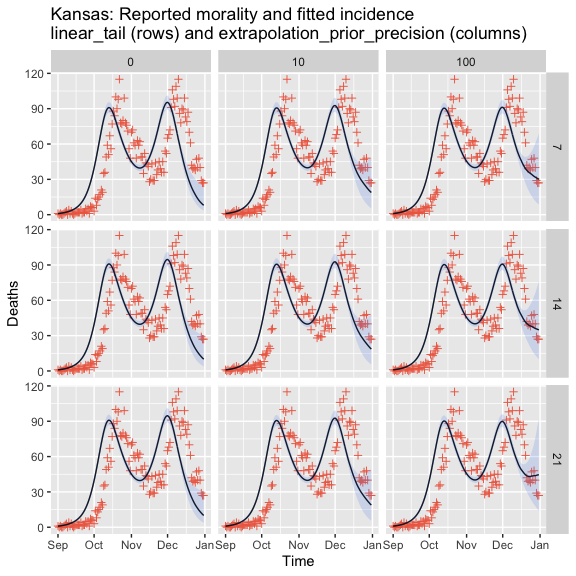The parameters are stable across a wide variety of settings for most data, but more care is needed when there is steep growth or decline at the point of truncation. ## Hyperparameter Tuning The empirical Bayes model fits a regularized likelihood model on a spline basis. There are two main hyperparameters: • degrees of freedom: the number of degrees of freedom in the spline basis; and • lambda: the regularization strength parameter. Each of these values are found by doing a sweep over a pre-specified set of possibilities. We can change those sets with the parameters: • dof_grid: a vector of possible degrees of freedom; and • lam_grid: a vector of possible lambda values. Note that these can be set as scalars to specify a fixed degree of freedom or lambda. Models output the selected degrees of freedom and lambda. Let’s look at the values for Kansas and change the grids.  kansas_model_hyperparameters = fit_incidence( reported = spanish_flu$Kansas,
delay_dist = delay_dist,
dof_grid = seq(22, 26, 2),
lam_grid = 10^(seq(1,-4, -1))
)
print(sprintf("Selected degrees of freedom from original model: %s; and updated model: %s",
as.character(kansas_model$best_dof), as.character(kansas_model_hyperparameters$best_dof)))
#>  "Selected degrees of freedom from original model: 20; and updated model: 22"
print(sprintf("Selected lambda from original model: %s; and updated model: %s",
as.character(kansas_model$best_lambda), as.character(kansas_model_hyperparameters$best_lambda)))
#>  "Selected lambda from original model: 0.00784759970351461; and updated model: 0.01"

plot(kansas_model_hyperparameters, times = spanish_flu$Date)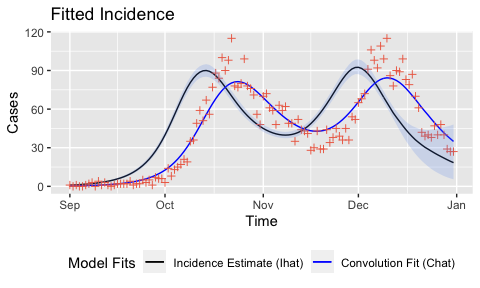These parameter settings are fairly robust, but larger data sets may need higher degrees of freedom or smaller lambda values. ## Hyperparameter Selection Methods For each hyperparameter, fit_incidence offers three ways of selecting the “best” value from the candidate set: • aic: Akaike information criterion; • bic: Bayesian information criterion; and • val: validation set likelihood. The first two methods are model-based optimism approximations, which run very quickly. The third involves randomized training/validation splits of the data to find which hyperparameter values maximize (up to a certain threshold) held out log likelihood. The default selection method is bic for degrees of freedom, and val for lambda. The methods can be changed with the dof_method and lam_method flags. Let’s fit the Kansas data with AIC for both hyperparameters. kansas_model_aic = fit_incidence( reported = spanish_flu$Kansas,
delay_dist = delay_dist,
dof_method = "aic",
lam_method = "aic"
)
print(sprintf("Selected degrees of freedom from original model: %s; and updated model: %s",
as.character(kansas_model$best_dof), as.character(kansas_model_aic$best_dof)))
#>  "Selected degrees of freedom from original model: 20; and updated model: 20"
print(sprintf("Selected lambda from original model: %s; and updated model: %s",
as.character(kansas_model$best_lambda), as.character(kansas_model_aic$best_lambda)))
#>  "Selected lambda from original model: 0.00784759970351461; and updated model: 5.45559478116853e-08"

plot(kansas_model_aic, times = spanish_flu$Date)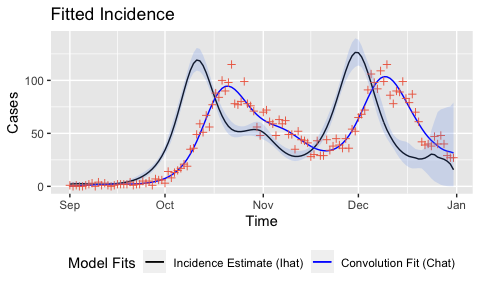AIC and BIC are reasonable selection methods for degrees of freedom, but can lead to overfitting when used for lambda selection. ## Validation Likelihood Hyperparameter Selection Validation likelihood breaks the data into a training and a validation set. For each potential hyperparameter value, a model is fit on the training set and and a held out log likelihood is computed on the validation set found by thinning. This yields a vector of validation log likelihoods, $$v_{1:d}$$, where $$d$$ is the number of candidate parameters. Blindly choosing the model with the highest held out log likelihood can lead to some overfitting on the validation set. Therefore, the validation method chooses the smallest degrees of freedom or largest lambda that produces a value within percent_thresh of the highest validation log likelihood. The hyperparameter index $$i \in \{1, \dots, d\}$$ is selected by: $\max \, i : \ \frac{\max(v) - v_i}{|\max(v)|} \leq \frac{\mathtt{percent\_thresh}}{100},$ where the indices are ordered from most regularization (lowest degrees of freedom or largest lambda) to least regularization. The default is percent_thesh = 2; lower values will generally give less regularized models while higher values will give more regularized models.  kansas_model_percent_thresh = fit_incidence( reported = spanish_flu$Kansas,
delay_dist = delay_dist,
percent_thresh = 0.2
)
print(sprintf("Selected degrees of freedom from original model: %s; and updated model: %s",
as.character(kansas_model$best_dof), as.character(kansas_model_percent_thresh$best_dof)))
#>  "Selected degrees of freedom from original model: 20; and updated model: 20"
print(sprintf("Selected lambda from original model: %s; and updated model: %s",
as.character(kansas_model$best_lambda), as.character(kansas_model_percent_thresh$best_lambda)))
#>  "Selected lambda from original model: 0.00784759970351461; and updated model: 0.000615848211066027"

plot(kansas_model_percent_thresh, times = spanish_flu\$Date)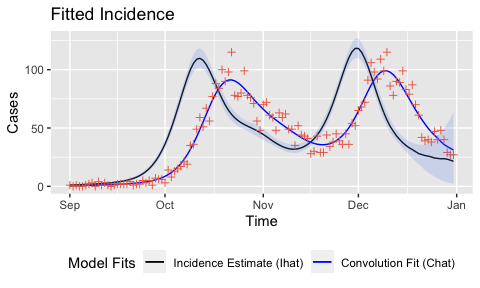The training/validation splits are random for this method, which can lead to instability if a single fit is used. Validation likelihood uses val_restarts fits, where the returned value is the average of the best lambdas or the median of the best degrees of freedom. Setting val_restarts to a lower value trades stability for speed.